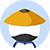What is the value of $a^{-2}b^{-3}$?

(1)$a^{-3}b^{-2}=36^{-1}$

(2)$(ab)^{-1}=6^{-1}$
• AStatement (1) ALONE is sufficient, but statement (2) alone is not sufficient.
• BStatement (2) ALONE is sufficient, but statement (1) alone is not sufficient.
• CBOTH statement TOGETHER are sufficient, but NEITHER statement ALONE is sufficient.
• DEACH statement ALONE is sufficient.
• EStatements (1) and (2) TOGETHER are NOT sufficient.

• 网友解析

题目讨论

• 优质讨论
• 最新讨论• 所属科目:数学DS
• 正确率: 74%
• 难度: